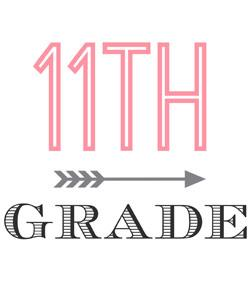# Grade 11 Accounting Review Trivia Quiz

10 Questions | Total Attempts: 5375SettingsThis is a brief quiz to review grade 11 accounting material.

• 1.
Which of the following is not a feature of the Balance Sheet?
• A.

Assets

• B.

Liabilities

• C.

Owner's Equity

• D.

Expenses

• 2.
A credit entry in the accounts would:
• A.

Decrease an asset

• B.

Increase a liability

• C.

Increase the capital account

• D.

All of above

• E.

B and c only

• 3.
The Trial Balance for a business is:
• A.

Another name for a balance sheet

• B.

A listing of account balances in a ledger to see if debits equal credits

• C.

A statement showing profit for the fiscal year

• D.

A check on the bank account balance

• 4.
Owner’s equity is best described as
• A.

The difference between the total assets and total liabilities of a business

• B.

A portion of total assets that has been bought using the owner’s money

• C.

The amount of inventory bought using the owner’s money

• D.

None of the above

• 5.
Assets are generally listed on a balance sheet in the order of their liquidity. Liquidity means:
• A.

The order in which the assets are converted into cash

• B.

Their dollar value, with the highest value listed first

• C.

Their dollar value, with the lowest value listed first

• D.

None of the above

• 6.
Which of the following is not a liability:
• A.

Mortgage on building

• B.

Bank loan on truck

• C.

Accounts payable

• D.

Accounts receivable

• 7.
Which of the following reflects the effect on the accounting equation of a purchase of an item of plant and equipment, for cash?
• A.

Assets increase: equity decreases

• B.

Assets decrease: equity increases

• C.

Assets decrease: equity unchanged

• D.

Assets unchanged: equity unchanged

• 8.
Which of the following reflects the effects on the accounting equation of a payment to creditors?
• A.

Assets decrease: ownership interest decreases

• B.

Assets decrease: ownership interest increases.

• C.

Assets decrease: liabilities decrease

• D.

Assets increase: liabilities decrease

• 9.
The fundamental accounting equation is usually expressed as:
• A.

Assets – liability = owner’s equity

• B.

Assets = liability – owner’s equity

• C.

Assets = liabilities + owners equity

• D.

Owner’s equity = assets = liability

• 10.
An Income Statement is:
• A.

A listing of the account balances in a ledger

• B.

A statement showing the financial position of a person, business or other organization

• C.

A financial statement that summarizes the items of revenue and expense, and shows, the net income or net loss of a business for a given period of time

• D.

All of the above

Related TopicsBack to top